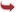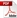International Journal of Scientific and Research Publications

#### IJSRP, Volume 2, Issue 12, December 2012 Edition [ISSN 2250-3153]Artificial Neural Network and Non-Linear Regression: A Comparative Study
Shraddha Srivastava, K.C. Tripathi
Abstract: Indian summer monsoon rainfall (ISMR) is an important metric to quantify the Asian monsoon system. Artificial Neural Networks, ANNs, are being increasingly used for nonlinear regression and classification problems in meteorology. The issues raised for this study can be summarized as the problem of simulation of the ISMR time series with the ANN model to get away with the need of external parameter for its prediction. The need of simulation with ANN needs to be justified by the same simulation being done by the linear and non-linear regression models. Time series analysis of the All India Rainfall Index using the ANN model and the linear and non-linear regression models are done. Lag Correlation analysis of the time series has been done to determine the predictors. The results are statistically analyzed to determine the quality of forecasts and also to do an inter-comparison of the models. Non-linear regression analysis was done to critically analyze the usefulness of the ANN models while modeling the AIR with the single data set. That the ANN model is a better option than the linear regression model was observed.
[VIEW FULL PAPER][DOWNLOAD]

Reference this Research Paper (copy & paste below code):

Shraddha Srivastava, K.C. Tripathi (2018); Artificial Neural Network and Non-Linear Regression: A Comparative Study; Int J Sci Res Publ 2(12) (ISSN: 2250-3153). http://www.ijsrp.org/research-paper-1212.php?rp=P12554
©️ Copyright 2011-2022 IJSRP - All rights reserved. Use of this web site signifies your agreement to the terms and conditions.